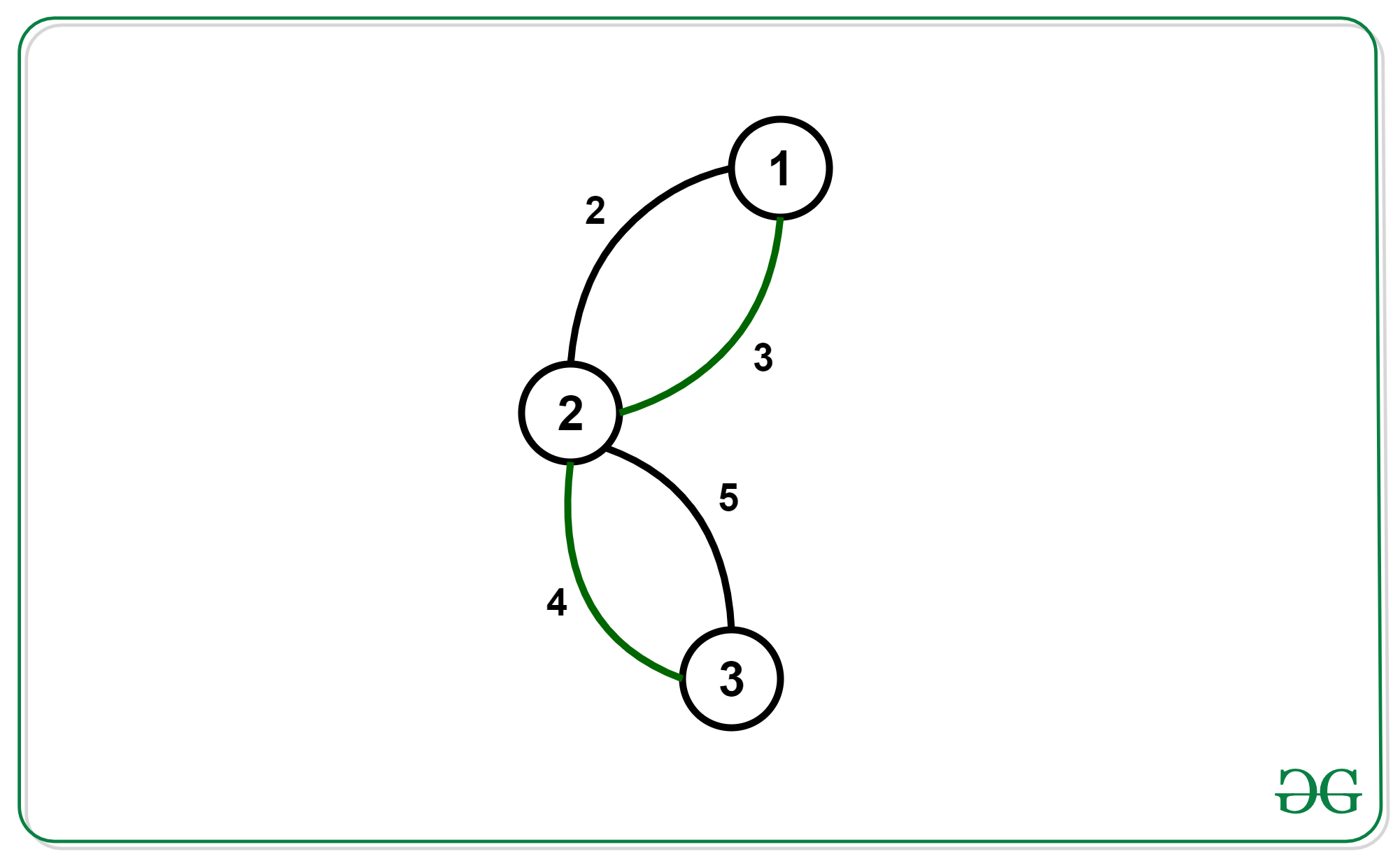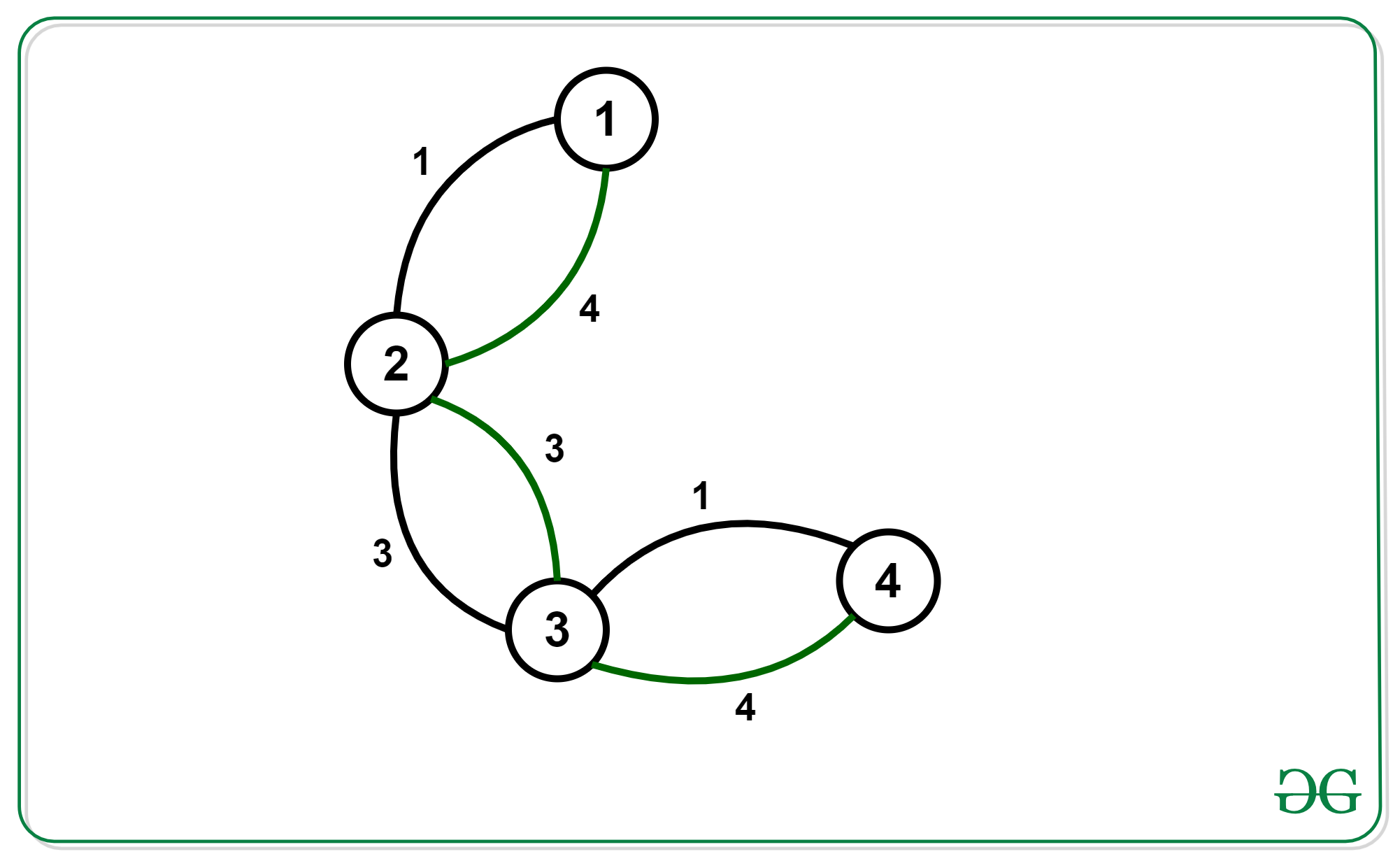Related Articles

# Find the minimum spanning tree with alternating colored edges

• Difficulty Level : Expert
• Last Updated : 19 Feb, 2020

Given a graph with N nodes and M edges where each edge has a color (either black or green) and a cost associated with it. Find the minimum spanning tree of the graph such that every path in the tree is made up of alternating colored edges.

Examples:

Input: N = 3, M = 4Output: 6

Input: N = 4, M = 6Output: 4

## Recommended: Please try your approach on {IDE} first, before moving on to the solution.

Approach:

• The first observation we make here is every such kind of spanning tree will be a chain. To prove it, suppose we have a tree that is not a chain and every path in it is made up of alternating edges. Then we can deduce that at least 1 node has a degree of 3. Out of these 3 edges, at least 2 will have the same color. The path using these 2 edges will never follow the conditions and Hence, such kind of tree is always a chain.
• Now we can find a chain with minimum cost and alternating edges using bitmask-dp,
dp[mask(2^n)][Node(n)][col_of_last_edge(2)] where the mask is the bitmask of nodes we’ve added to the chain. Node is the last node we added to the chain.col_of_last_edge is the color of edge use to connect Node.
• To transition from 1 state to another state we visit the adjacency list of the last node and use those edges which have color != col_of_last_edge.

Below is the implementation of the above approach:

## C++

 `// C++ program for the``// above approach``#include ``using` `namespace` `std;`` ` `int` `graph;`` ` `// Initializing dp of size =``// (2^18)*18*2.``long` `long` `dp[1 << 18];`` ` `// Recursive Function to calculate``// Minimum Cost with alternate ``// colour edges``long` `long` `minCost(``int` `n, ``int` `m, ``int` `mask, ``int` `prev, ``int` `col)``{``    ``// Base case``    ``if` `(mask == ((1 << n) - 1)) {``        ``return` `0;``    ``}``    ``// If already calculated``    ``if` `(dp[mask][prev][col == 1] != 0) {``        ``return` `dp[mask][prev][col == 1];``    ``}`` ` `    ``long` `long` `ans = 1e9;`` ` `    ``for` `(``int` `i = 0; i < n; i++) {``        ``for` `(``int` `j = 0; j < 2; j++) {``            ``// Masking previous edges``            ``// as explained in above formula.``            ``if` `(graph[prev][i][j] && !(mask & (1 << i)) ``                ``&& (j != col)) {``                ``long` `long` `z = graph[prev][i][j] + ``                              ``minCost(n,m,mask|(1<,``                      ``pair<``int``,``char``>>>& vp,``int` `m){`` ` `  ``for` `(``int` `i = 0; i < m; i++) {``    ``int` `a = vp[i].first.first - 1;``    ``int` `b = vp[i].first.second - 1;``    ``int` `cost = vp[i].second.first;``    ``char` `color = vp[i].second.second;``    ``graph[a][b][color == ``'W'``] = cost;``    ``graph[b][a][color == ``'W'``] = cost;``  ``}``}`` ` `// Function to getCost``// for the Minimum Spanning``// Tree Formed``int` `getCost(``int` `n,``int` `m){``     ` `    ``// Assigning maximum``    ``// possible value.``    ``long` `long` `ans = 1e9;`` ` `    ``for` `(``int` `i = 0; i < n; i++) {``        ``ans = min(ans, minCost(n, m, 1 << i, i, 2));``    ``}`` ` `    ``if` `(ans != 1e9) {``        ``return` `ans;``    ``}``    ``else` `{``        ``return` `-1;``    ``}``}`` ` `// Driver code``int` `main()``{``    ``int` `n = 3, m = 4;``    ``vector, pair<``int``, ``char``> > > vp = {``        ``{ { 1, 2 }, { 2, ``'B'` `} },``        ``{ { 1, 2 }, { 3, ``'W'` `} },``        ``{ { 2, 3 }, { 4, ``'W'` `} },``        ``{ { 2, 3 }, { 5, ``'B'` `} }``    ``};`` ` `    ``makeGraph(vp,m);``    ``cout << getCost(n,m) << ``'\n'``;``     ` `    ``return` `0;``}`

## Python3

 `# Python implementation of the approach`` ` `graph ``=` `[[[``0``, ``0``] ``for` `i ``in` `range``(``18``)] ``for` `j ``in` `range``(``18``)]`` ` `# Initializing dp of size =``# (2^18)*18*2.``dp ``=` `[[[``0``, ``0``] ``for` `i ``in` `range``(``18``)] ``for` `j ``in` `range``(``1` `<< ``15``)]`` ` `# Recursive Function to calculate``# Minimum Cost with alternate``# colour edges``def` `minCost(n: ``int``, m: ``int``, mask: ``            ``int``, prev: ``int``, col: ``int``) ``-``> ``int``:``    ``global` `dp`` ` `    ``# Base case``    ``if` `mask ``=``=` `((``1` `<< n) ``-` `1``):``        ``return` `0`` ` `    ``# If already calculated``    ``if` `dp[mask][prev][col ``=``=` `1``] !``=` `0``:``        ``return` `dp[mask][prev][col ``=``=` `1``]`` ` `    ``ans ``=` `int``(``1e9``)`` ` `    ``for` `i ``in` `range``(n):``        ``for` `j ``in` `range``(``2``):`` ` `            ``# Masking previous edges``            ``# as explained in above formula.``            ``if` `graph[prev][i][j] ``and` `not` `(mask & (``1` `<< i)) \``                ``and` `(j !``=` `col):``                ``z ``=` `graph[prev][i][j] ``+` `minCost(n,``                        ``m, mask | (``1` `<< i), i, j)``                ``ans ``=` `min``(z, ans)`` ` `    ``dp[mask][prev][col ``=``=` `1``] ``=` `ans``    ``return` `dp[mask][prev][col ``=``=` `1``]`` ` `# Function to Adjacency``# List Representation``# of a Graph``def` `makeGraph(vp: ``list``, m: ``int``):``    ``global` `graph``    ``for` `i ``in` `range``(m):``        ``a ``=` `vp[i][``0``][``0``] ``-` `1``        ``b ``=` `vp[i][``0``][``1``] ``-` `1``        ``cost ``=` `vp[i][``1``][``0``]``        ``color ``=` `vp[i][``1``][``1``]``        ``graph[a][b][color ``=``=` `'W'``] ``=` `cost``        ``graph[b][a][color ``=``=` `'W'``] ``=` `cost`` ` `# Function to getCost``# for the Minimum Spanning``# Tree Formed``def` `getCost(n: ``int``, m: ``int``) ``-``> ``int``:`` ` `    ``# Assigning maximum``    ``# possible value.``    ``ans ``=` `int``(``1e9``)``    ``for` `i ``in` `range``(n):``        ``ans ``=` `min``(ans, minCost(n, m, ``1` `<< i, i, ``2``))`` ` `    ``if` `ans !``=` `int``(``1e9``):``        ``return` `ans``    ``else``:``        ``return` `-``1`` ` `# Driver Code``if` `__name__ ``=``=` `"__main__"``:`` ` `    ``n ``=` `3``    ``m ``=` `4``    ``vp ``=` `[[[``1``, ``2``], [``2``, ``'B'``]],``        ``[[``1``, ``2``], [``3``, ``'W'``]],``        ``[[``2``, ``3``], [``4``, ``'W'``]],``        ``[[``2``, ``3``], [``5``, ``'B'``]]]``    ``makeGraph(vp, m)``    ``print``(getCost(n, m))`` ` `# This code is contributed by``# sanjeev2552`
Output:
```6
```

Time Complexity: O(2^N * (M + N))My Personal Notes arrow_drop_up